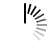# Polynomial Time Approximation Scheme

#### Polynomial Time Approximation scheme

We can find some polynomial time solution for NP-Complete problems like 0-1 Knapsack problem or Subset sum problem. These problems are very popular in the real world, so there must be some ways to handle these problems.

The Polynomial Time Approximation Scheme (PTAS) is a type to approximate algorithms for optimization problems. For the 0-1 Knapsack problem, there is a Pseudo Polynomial Solution, but when the values are large, the solution is not feasible. Then we need a PTAS solution.

Some NP-complete problems like Graph Coloring, K-Center problem etc. they have no known polynomial time solution. PTAS used to approximate the algorithms. These algorithms take a parameter ε> 0 and to approximate we will minimize (1 + ε) and maximize (1 - ε).

#### Example

As an example, if we choose a minimization problem and take ε = 0.5, then the solution by using the PTAS is nearly 1.5. So the running time must be polynomial in terms of n, but it can be exponential in terms of ε.